# SSAT Upper Level Math : How to divide complex fractions

## Example Questions

2 Next →

### Example Question #11 : How To Divide Complex Fractions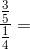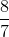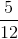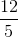Explanation:

The main line in a fraction means "divide." Rewrite the complex fraction like this: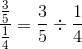Now, divide these two fractions.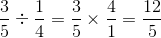### Example Question #12 : How To Divide Complex Fractions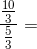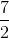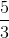Explanation:

The main fraction line means "divide." Rewrite the complex fraction like this: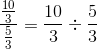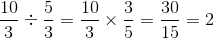### Example Question #13 : How To Divide Complex Fractions

Simplify: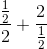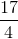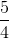Explanation:

Rewrite the expression using a division sign.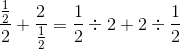Take the reciprocal of the term after the division signs and convert the division sign to a multiplication sign.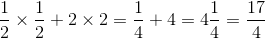2 Next →